# 逻辑回归与正则化

Posted by jjx on December 3, 2016

#### 假说表示

X 代表特征向量 g 代表逻辑函数(logistic function)是一个常用的逻辑函数为 S 形函数(Sigmoid function),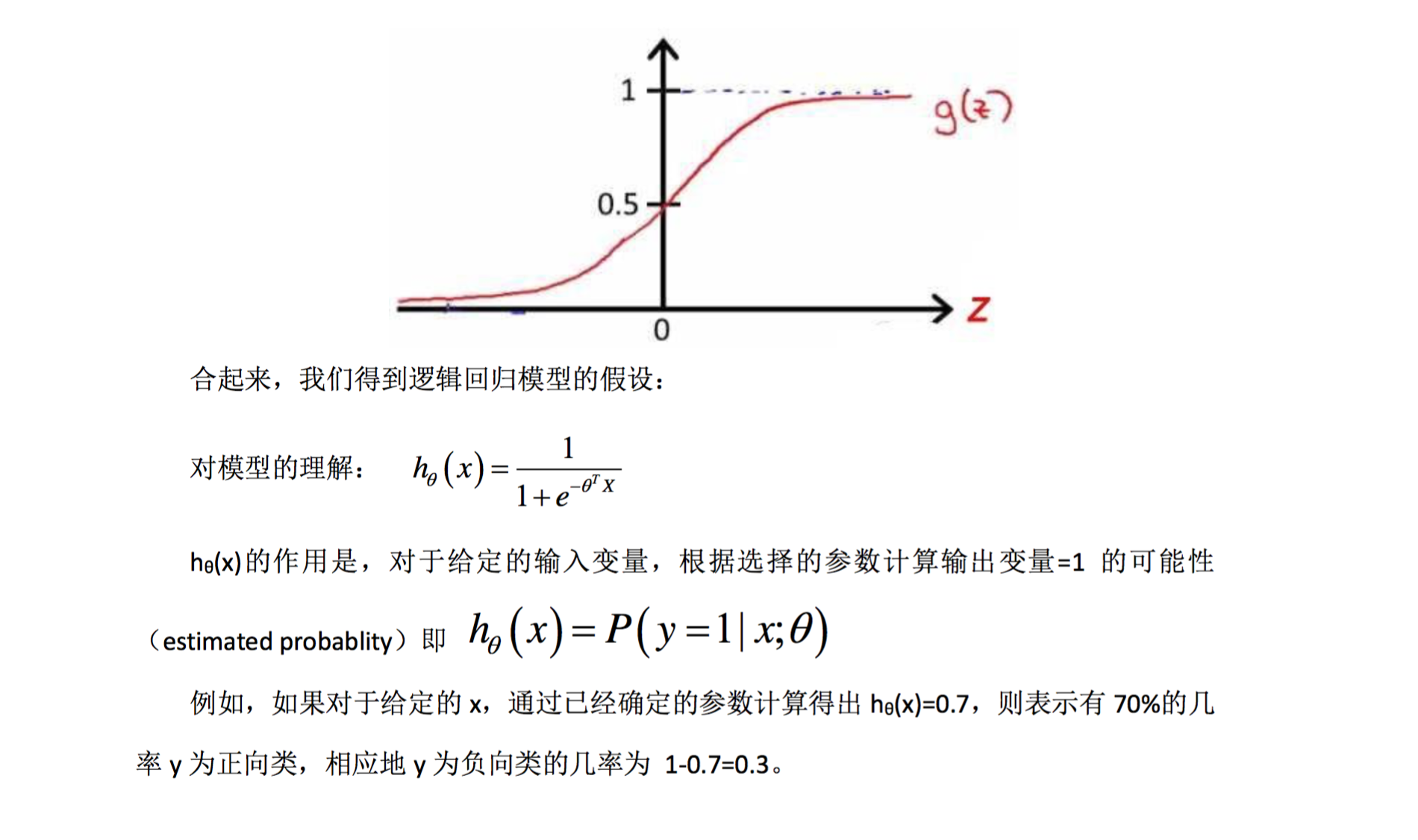z>0 时 g(z)>0.5
z<0 时 g(z)<0.5

$θ^TX$ 大于等于 0 时,预测 y=1
$θ^TX$ 小于 0 时,预测 y=0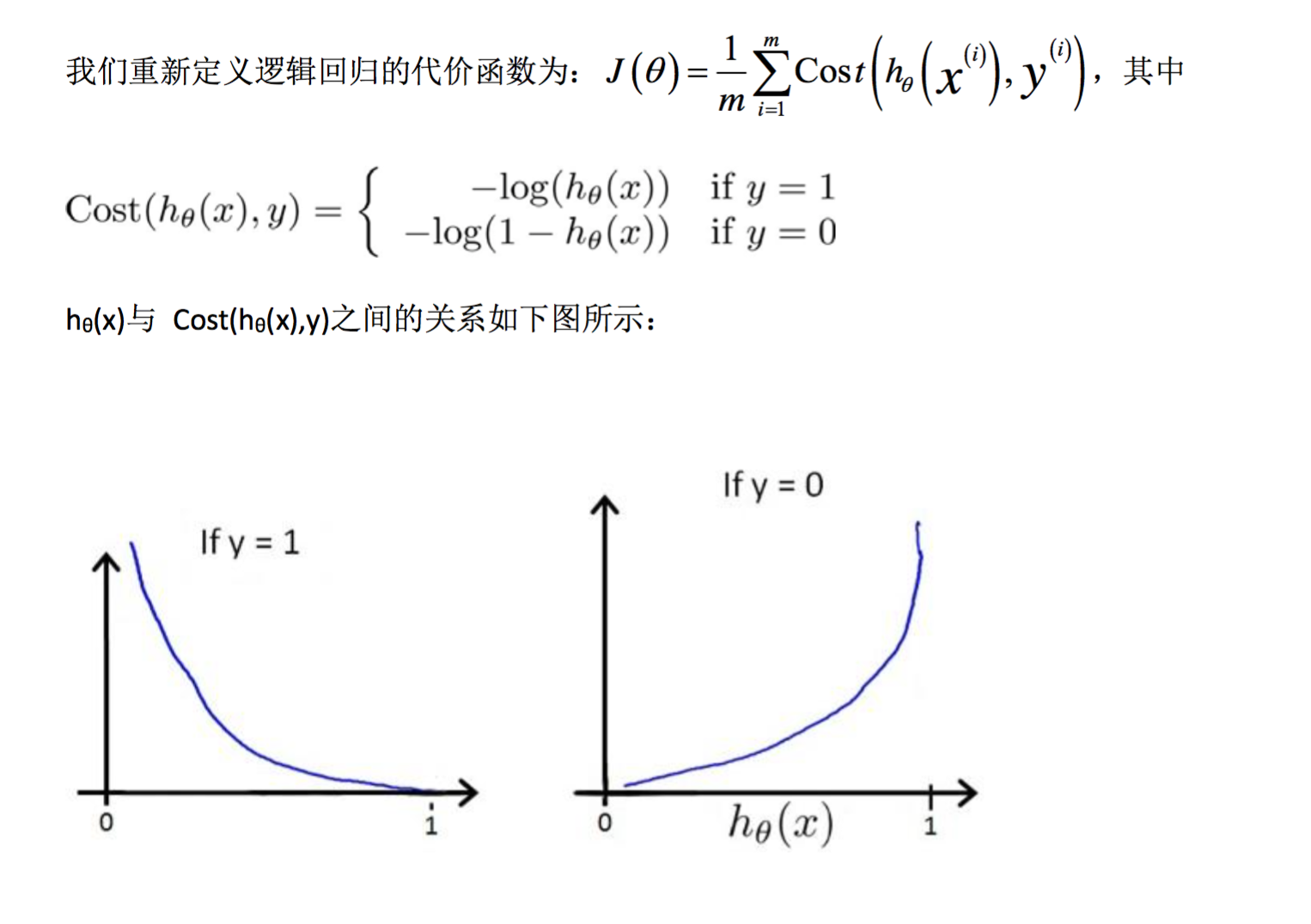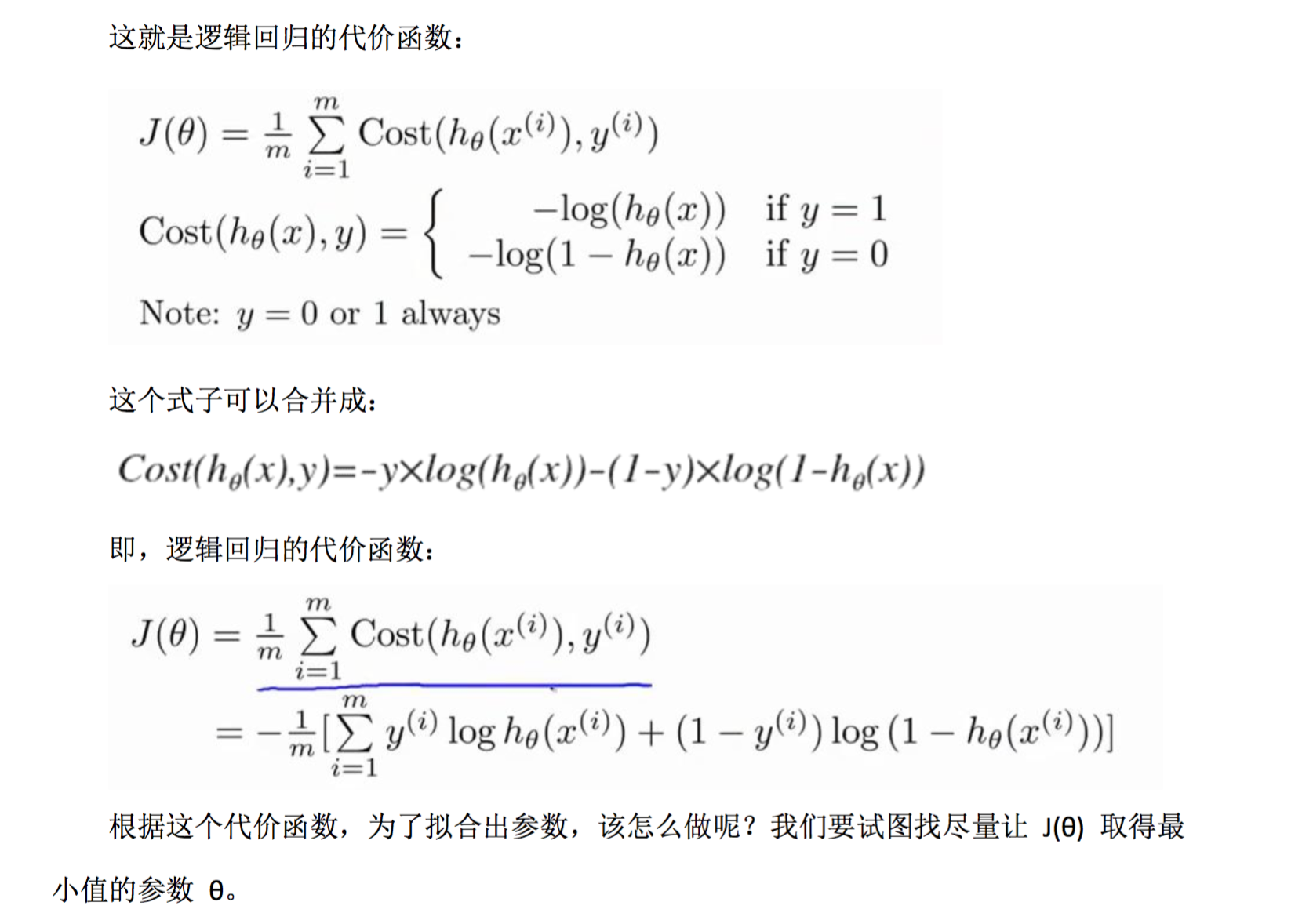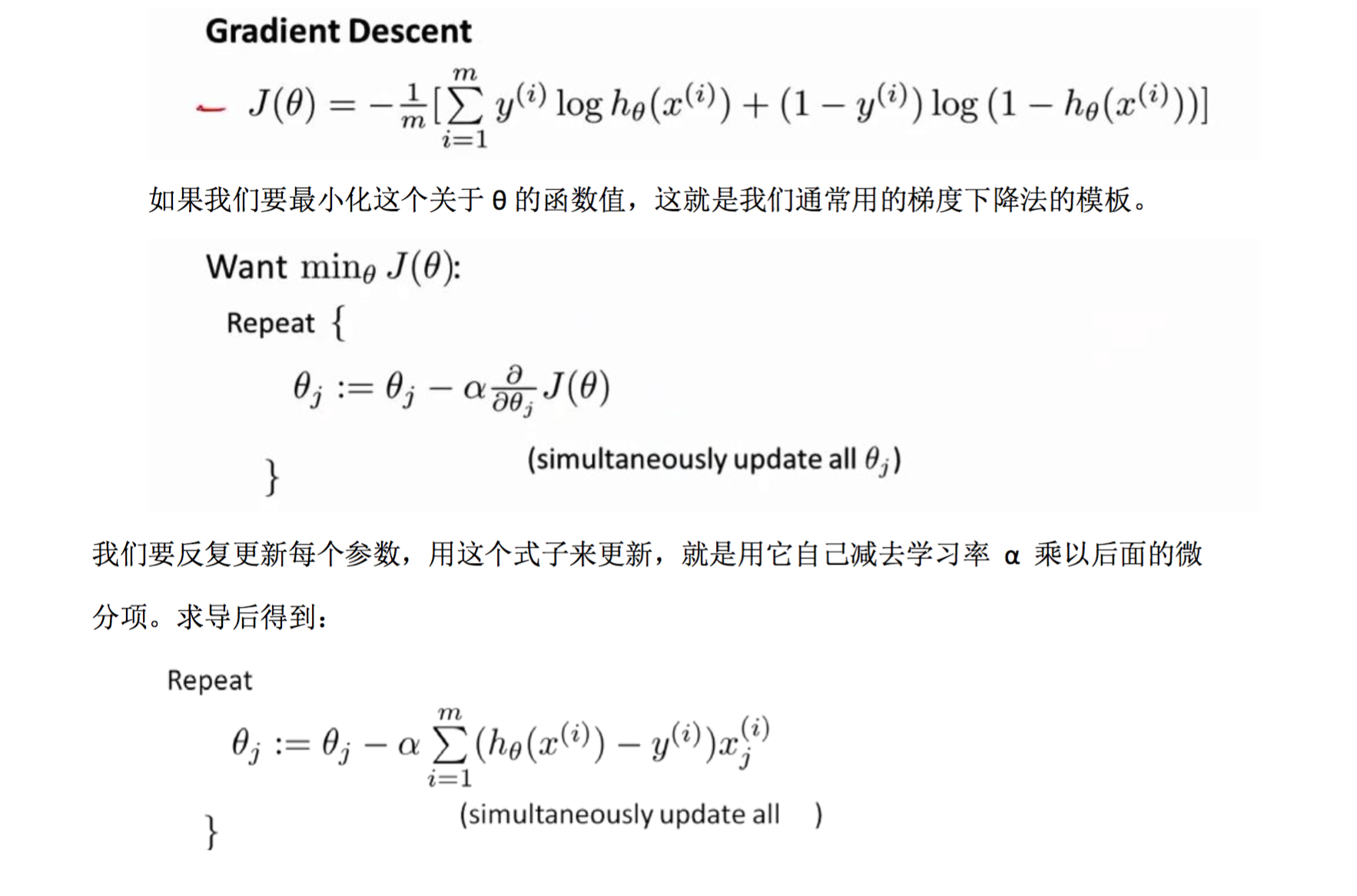#### 高级优化

• 共轭梯度法
• BFGS (变尺度法)
• L-BFGS (限制变尺 度法)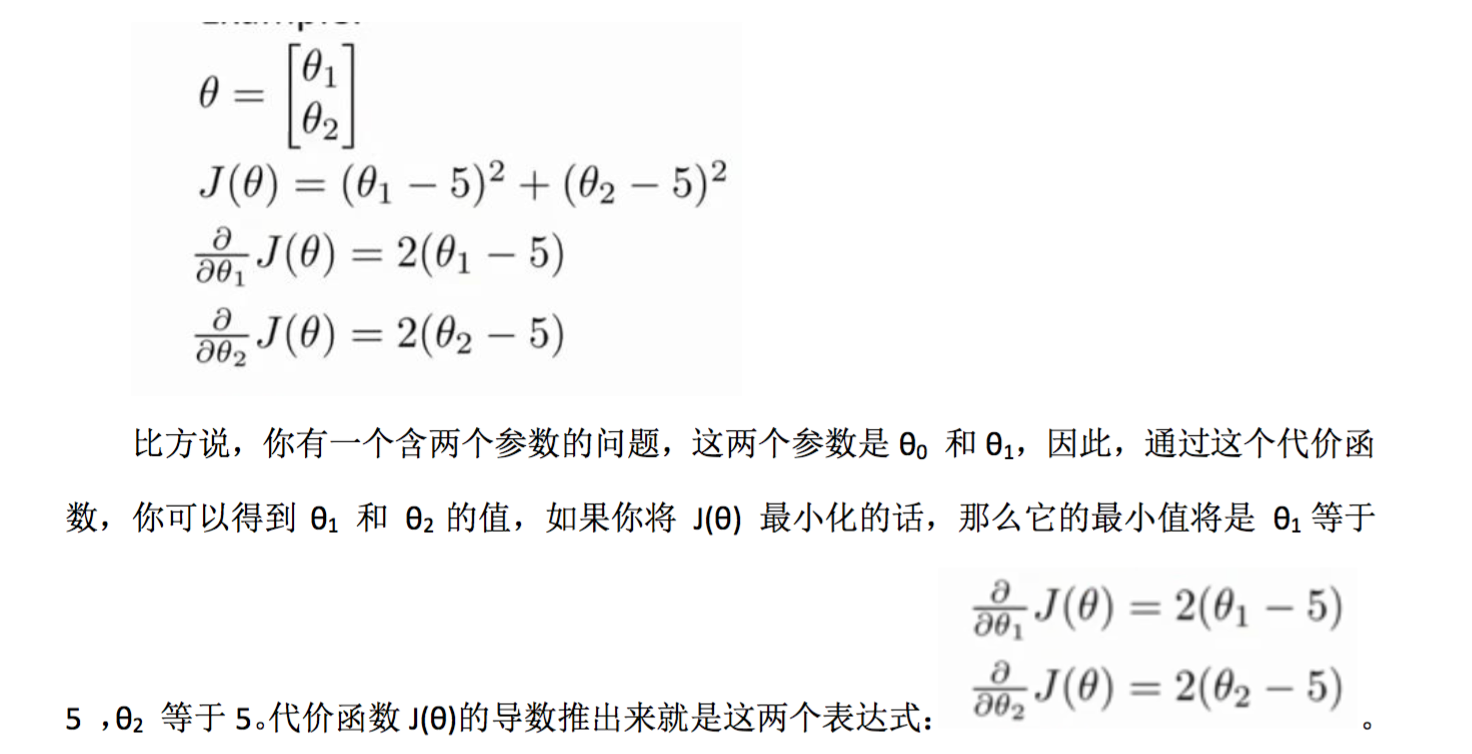function [jVal, gradient]=costFunction(theta)
jVal=(theta(1)-5)^2+(theta(2)-5)^2;
end


options=optimset('GradObj','on','MaxIter',100);
initialTheta=zeros(2,1);
[optTheta, functionVal, exitFlag]=fminunc(@costFunction, initialTheta, options);


#### 多类别分类:一对多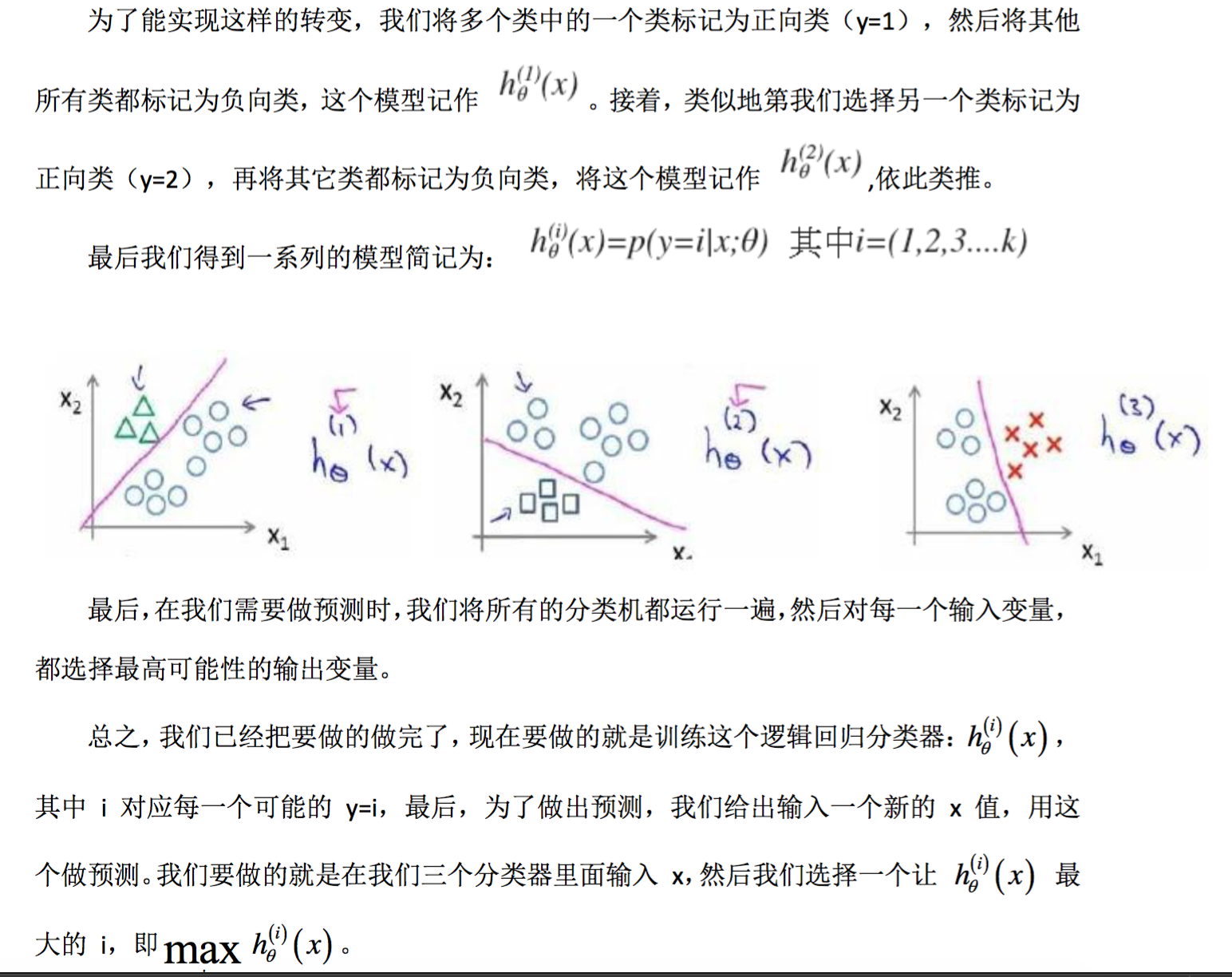#### 正则化(Regularization)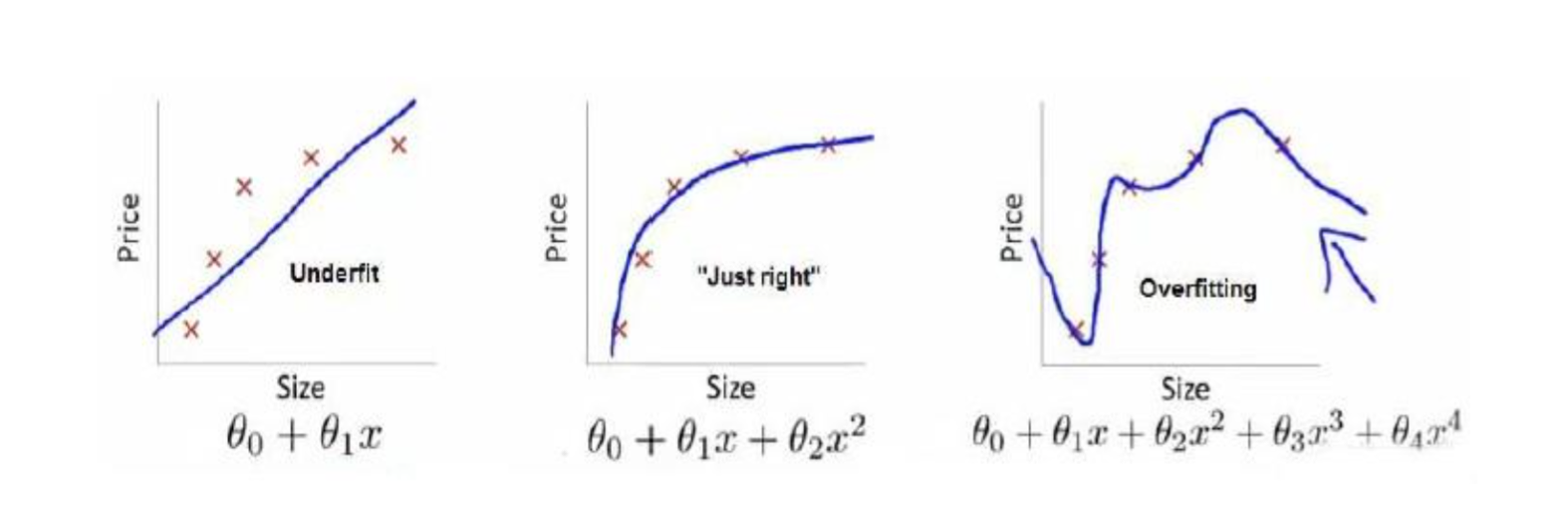1. 丢弃一些不能帮助我们正确预测的特征。可以是手工选择保留哪些特征,或者使用 一些模型选择的算法来帮忙(例如 PCA)
2. 正则化。 保留所有的特征,但是减少参数的大小(magnitude)。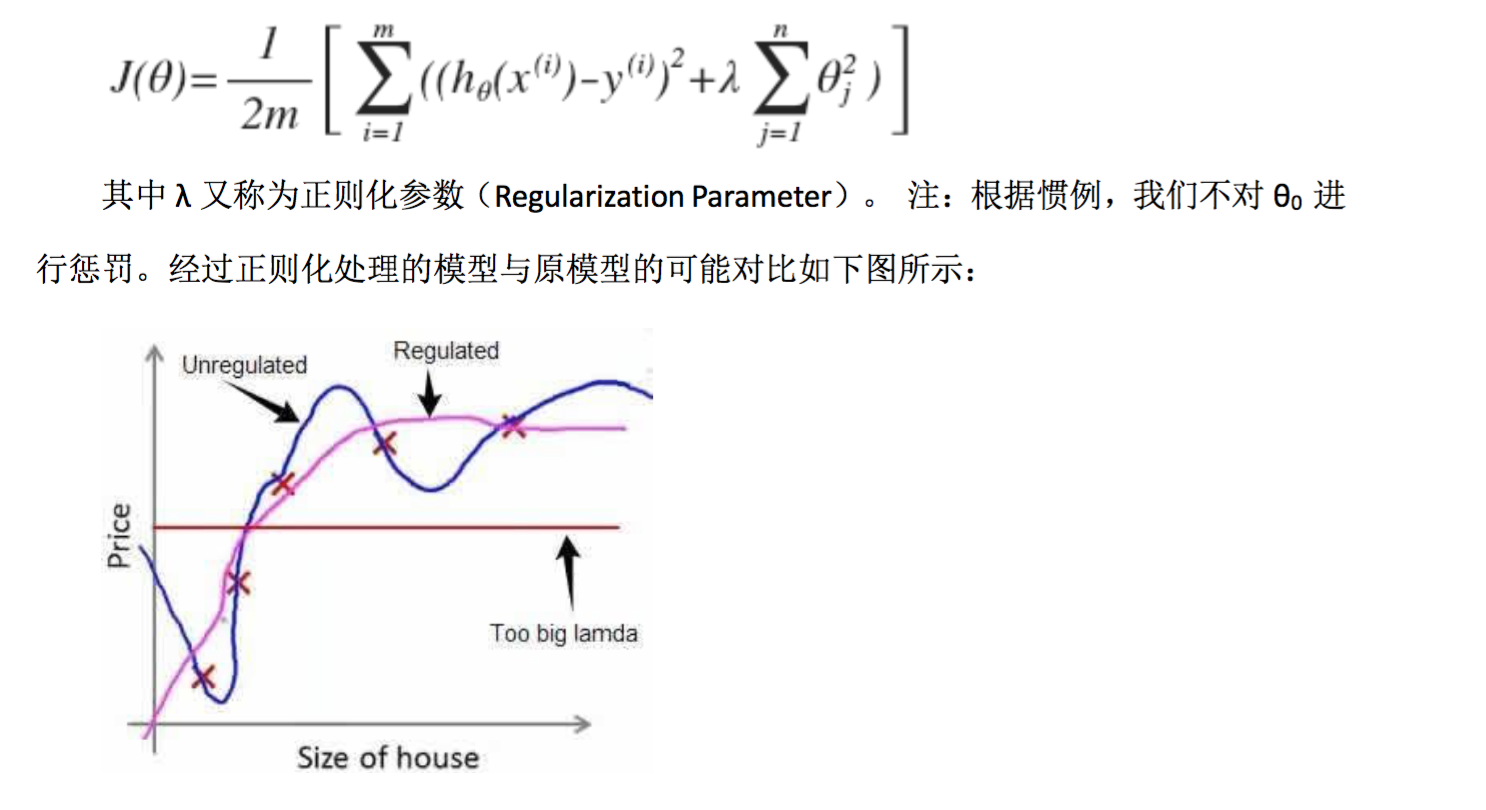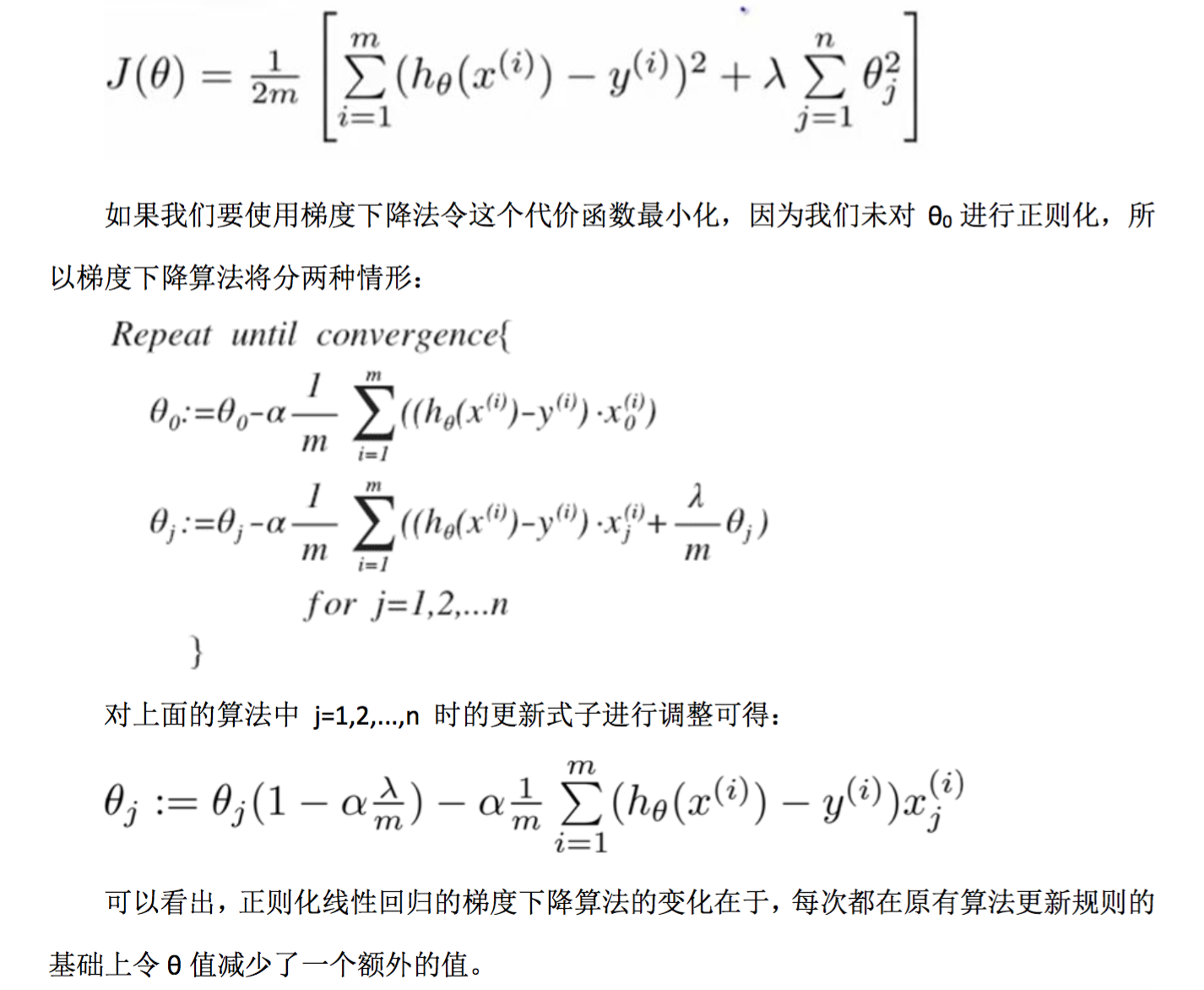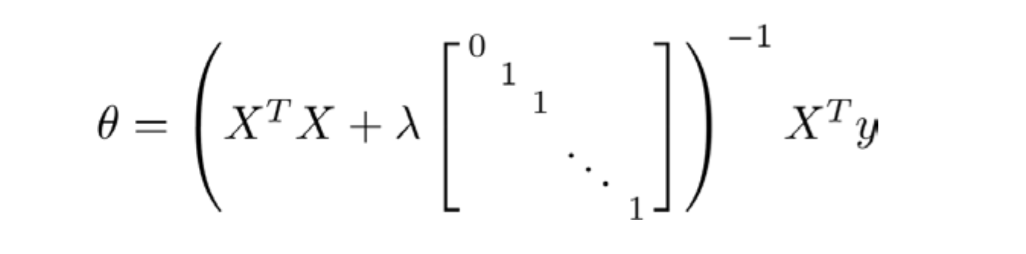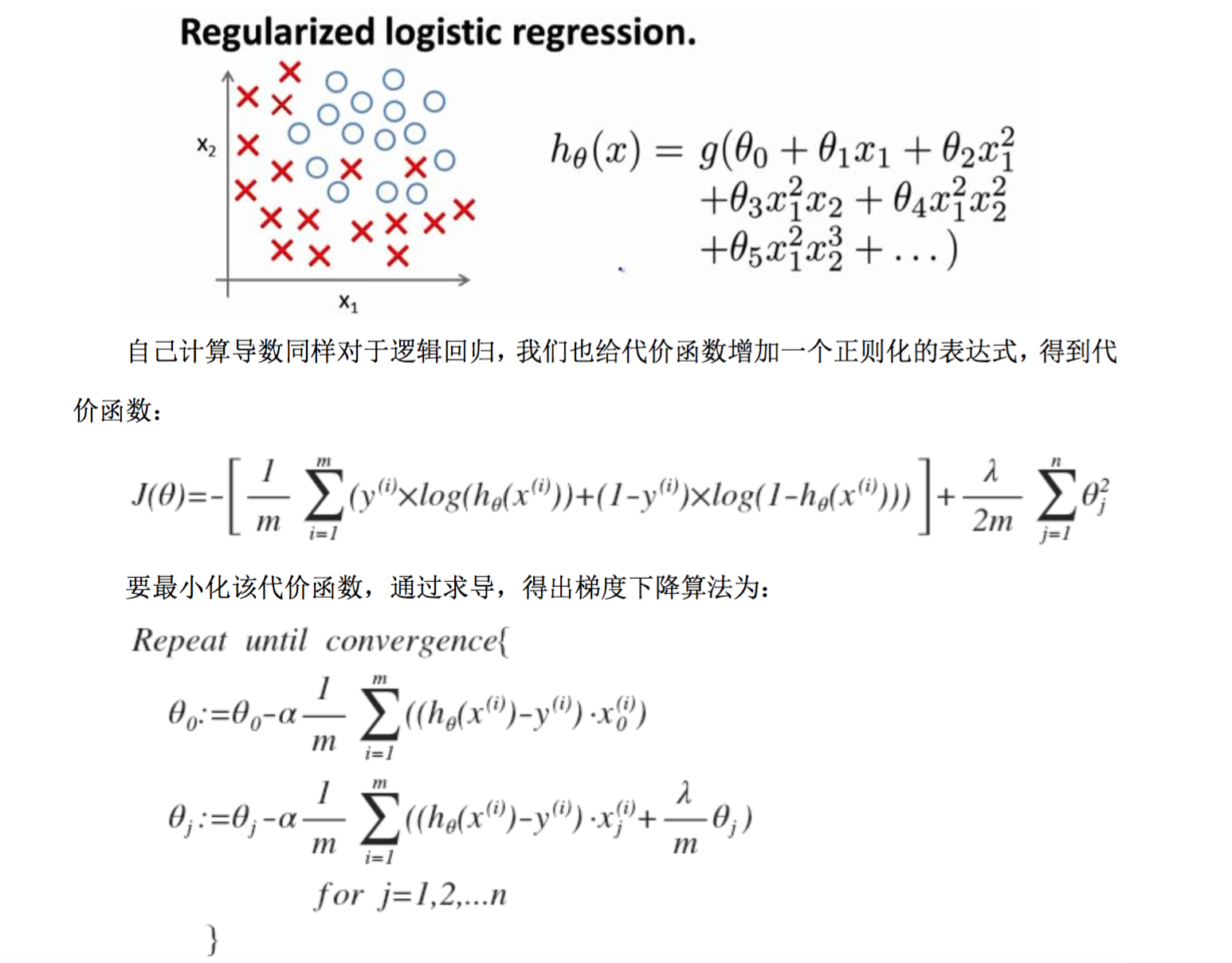1.虽然正则化的逻辑回归中的梯度下降和正则化的线性回归中的表达式看起来一样,但 由于两者的 h(x)不同所以还是有很大差别。

1. θ0 不参与其中的任何一个正则化。